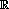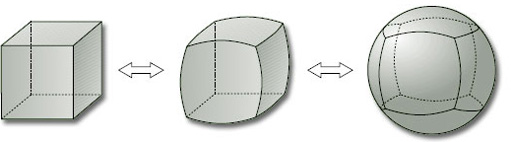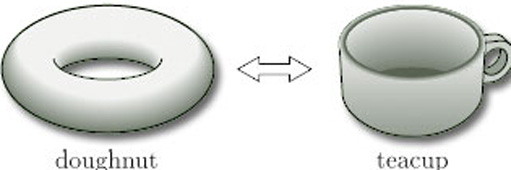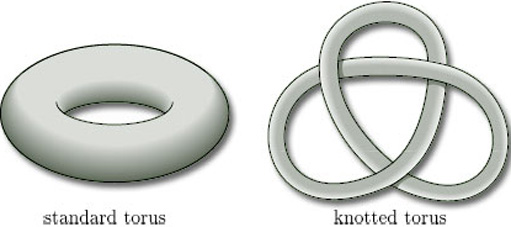Surfaces

Start this free course now. Just create an account and sign in. Enrol and complete the course for a free statement of participation or digital badge if available.

Free course

# 2.4 Homeomorphic surfaces

As we stated in Section 1, our aim is to classify surfaces up to homeomorphism. So it is worthwhile spending a little time examining what sorts of transformations of surfaces are homeomorphisms. We shall restrict the description to surfaces in space, as these are easier to deal with, though the result at the end of this subsection applies to all surfaces.

Recall that a homeomorphism between two topological spaces (such as surfaces in space) is a bijection with the property that both f and its inverse f−1 preserve open sets.

Roughly speaking, homeomorphisms between surfaces in space are of two kinds. One kind includes any transformation of a surface in space that can be achieved by bending, stretching, squeezing or shrinking the surface. These maps are certainly one–one and onto, and (intuitively, at least) seem to be continuous – and thus are bijections. They would also seem to preserve open sets, if we remember that the topology of a surface in space is the subspace topology inherited from3. So, essentially, we can achieve a homeomorphism by treating a surface in space as if it were made from a sheet of rubber and then bending, stretching, squeezing or shrinking it. Topology is sometimes called rubber-sheet geometry.

For example, the sphere in its usual round form and in various lumpy forms (such as a cube) are homeomorphic, as we can see by pumping air into the lumpy one and inflating it as if it were a balloon, as illustrated in Figure 39. If the surface has sharp edges and vertices, then stretching and smoothing it can remove these edges and vertices.Figure 39 Inflating a cube

This sort of homeomorphism also explains why we are able to draw n-fold toruses either in doughnut form or in the form of a sphere with handles – both representations are topologically equivalent. It also explains the description of a topologist as someone who doesn't know the difference between a doughnut and a teacup, since both shapes are topologically equivalent under a homeomorphism of this kind (Figure 40).Figure 40

As an example of the other kind of homeomorphism, consider the torus in its usual form, sometimes referred to as its standard form, and in a knotted form, as shown in Figure 41.Figure 41

It is impossible to ‘unknot’ the knotted torus just by bending, stretching, squeezing or shrinking it – but we can cut it and open it out, rearrange it as a cut torus, and then glue it back together, as shown in Figure 42.

Provided that we are able to join the points on either side of the cut exactly as they were beforehand, the whole operation defines a homeomorphism between the two surfaces. If this seems surprising, look at the neighbourhood of a point on the cut, as enlarged in Figure 43: clearly, open discs are mapped to open discs without any change.

To sum up, two surfaces in space are homeomorphic if we can bend, stretch, squeeze or shrink one into the other and/or if we can cut one and then, after some bending, stretching, squeezing or shrinking, glue it back together (making sure to join the points on either side of the cut exactly as beforehand) to form the other. We thus have a practical and intuitive way of demonstrating that surfaces in space are homeomorphic.

There are also practical ways of showing that two surfaces in space are not homeomorphic. One is to consider the boundary number of the surface.

## Theorem 2

Two surfaces that are homeomorphic have the same boundary number.

(We omit the proof, which is beyond the scope of this course.)

M338_1